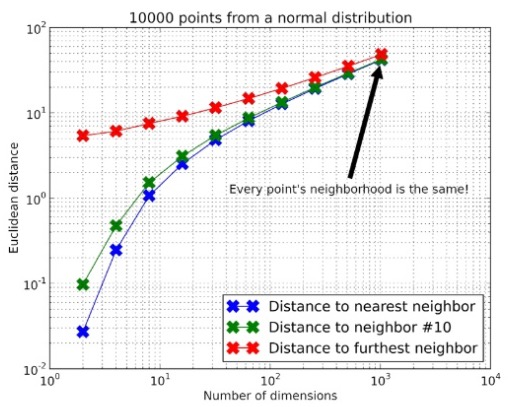# Locality-sensitive hashing (LSH)

Alex Egg,

In order to preform nearest neighbour search (NN) on large and high dimensional datasets you must overcome 2 big problems:

1. ineffectiveness of distance measures in high dimensions
2. computational intractability of exhaustive search on large datasets

By employing hash tables w/ a novel hashing function that optimises for collisions we reduce the search space and mitigate the curse of dimensionality for NN search.Figure 1: The curse of dimensionality visualized: In high dimensions, every point is equally far away!.

## Hash Function

Given a vector $x$ in $\mathbb{R^d}$ and a projection matrix $\mathrm{P}$ in $\mathbb{R^{r\times d}}$ we can formulate a hashing function $h(x)$:

where $h(x)$ is a vector in the $\mathbb{R^r}$ space and $\mathrm{P}$ is random matrix parameterised by $r$ $(r\ll d)$.

The key here is that $x$ is referenced in a smaller dimension than before $r$ vs $d$ .

## Hash Table

Given a hashing function that maximises collisions and a dictionary data structure we can build a hash table $D$:

where $D$ is a mapping of $h(x)$ to $[x_1,…,x_n]$ where each $x$ has a degree of similarity.

Formally, we can find $x$ in $D$ in $O(1)$ compared to $O(n)$ for exhaustive search.

## Nearest Neighbour

In order to preform NN in the pathological case, it would be $O(n)$ search w/ no upper bound on $n$. However, in the LSH case, we can put an upper bound on $n$ and guarantee that: $n_{LSH}\ll n_{NN}$ as we are guaranteed to never have to iterate the whole search space:

1. Reduce search space by querying $\ell \leftarrow D : h(x)$
2. Preform classical NN search on the drastically reduced search space $\ell$ where the upper bound on $n$ is $\ell$

## Conclusion

In order to preform NN on large and high dimensional datasets you must overcome 2 big problems:

1. ineffectiveness of distance measures in high dimensions
2. computational intractability of exhaustive search on large datasets

By employing hash tables w/ a novel hashing function that optimises for collisions we reduce the search space and mitigate the curse of dimensionality for NN search.